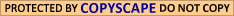# GPS System## How does a GPS system work?

A GPS system works by using precise calculations to determine its location in relation to the GPS satellites orbiting the earth. Receivers are able to determine their position by combining time, distance and velocity measurements in a mathematical calculation known as triangulation.

GPS system receivers and GPS satellites communicate via Pseudo-Random Code, a complex combination of digital radio pulses. Satellite and receiver pulses are deemed to be in perfect synch for the purpose of calculating distance. Each satellite emits its own unique pseudo-random code that is picked up by the receiver in a GPS system and compared to its own code.

GPS satellites are fitted with extremely precise atomic clocks that allow them to record exact universal time. By contrast, GPS receivers are fitted with common quartz clocks. A receiver calculates its distance from a satellite by measuring the difference in time between the emission of its own pseudo-random pulses and those acquired from the satellite. It then multiplies this time difference by the speed of light which is approximately186, 000 miles per second. The receiver can then calculate how far it is from a particular satellite.

If receivers were able to keep precise universal time, the pseudo-random emissions from all satellites would intercept at a single point and three satellite readings would be sufficient to determine exact distance calculations. But because receivers only use run of the mill quartz clocks, provision needs to be made for inconsistencies in the time kept by the receiver and the time kept by the satellites. Receivers therefore require emissions from a minimum of four satellites in order to calculate the exact distances of satellites. Receivers use the readings from the fourth satellite to check the accuracy of their own clocks.

While the emissions from the first three satellites intercept at a single point, the emission from the fourth will not intercept with the other three due to the fractional inaccuracy in time kept by receivers. Receivers use the emission from the fourth satellite to correct any discrepancy in their timing calculations and reset their clocks to the precise universal time as kept by the satellites. This will enable it to determine a distance measurement that is completely accurate.

Distance calculations are combined with almanac data that is received from a satellite in conjunction with pseudo-random code emissions. Almanac data contains the exact orbital position of a satellite as well as its position in relation to other satellites in a constellation. Slight variations in satellite position are monitored by the United Stated Department of Defense, so that when an inaccuracy occurs an ephemeris error signal can be transmitted to a satellite and its orbital position can be corrected.

Other factors such as the concentration of particles in the earth's atmosphere and slight discrepancies in atomic clock readings may also lead to errors in receiver calculations. Differential GPS eliminates these errors by combining the readings recorded by a stationary receiver at a reference station with those received by a moving receiver in a GPS system. The stationary receiver uses its position to calculate the timing of signals. The difference between the expected travel time of satellite signals and the actual travel time is then calculated as an error reading.

The stationary receiver relays this information to moving receivers in its vicinity. A moving receiver acquires information on all satellite signal errors and can account for any errors that apply to the specific satellites it is utilizing. A receiver is therefore able to combine precise distance and positional information gained from satellites with error information it obtains from a reference receiver to calculate its own exact position on earth.GPS Systems Online

GPS Systems

GPS Devices
GPS Devices
GPS Reviews Online
Discount GPS

GPS Technology
GPS System
GPS Tracking System Online